### On Isogenies Verifiable Delay Functions (VDF)

This continues the post from part 1.

In the previous post we discussed about Verifiable Delay Functions. Here is a quick summary of what we discussed in the first part:
• We covered the definition of  Verifiable Delay Functions (VDF).
• We have seen a short history of how this idea slowly developed.
• We hinted the existence of some constructions.
• We described some application with an eye on the blockchain World.
In this post we will switch gear and we will focus on a particular construction: the isogenies VDF (a construction defined by Luca De Feo, Simon Masson, Christophe Petit and myself). We try to keep the description as easy as possible simplifying many things, without trying not to worry too much about mathematical rigor (that risks to become mathematical rigor mortis :)), so mathematicians out there I beg your pardon! .

## Isoge WHAT?

Isogeny based cryptography is last baby in the (cryptography) family. Below you can see a short history of isogeny based cryptography.History of isogeny-based cryptography

As matter of fact, the SIDH paper ignited the field and several papers where published after it. But, before to put our attention on isogenies, let's take a step back toward elliptic curve cryptography.

### Elliptic Curves

With a lot of simplification, an elliptic curve is the set of solutions defined by an equation of the form

y² = x3+ ax + b

Equations of this type are called Weierstrass equations. An elliptic curve would look like: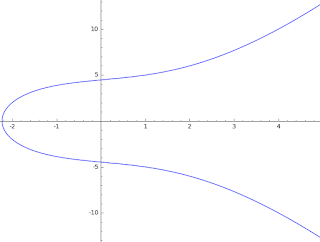y² = x3 + 4x + 20

In order to apply the theory of elliptic curves to cryptography we need to look at elliptic curves whose points have coordinates in a finite field Fq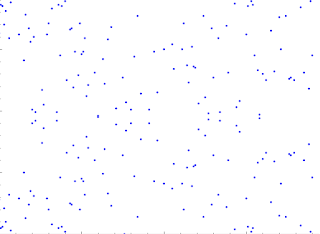y² = x3 + 4x + 20 over Finite Field of size 191
To start doing cryptography with elliptic curves you need a given curve (e.g. NIST curve as P-256 or DJB's Curve 25519) and a fixed base point in the curve. Now to build any crypto system based on elliptic curve we need some operation to work with.
So here some geometric intuition of how we define points addition on a given elliptic curve. Given two points P and Q, you draw a line (and given the fact the equation is cubic) the line will hit a curve on a third point R, then you take the reflection of it -R and this defines the result.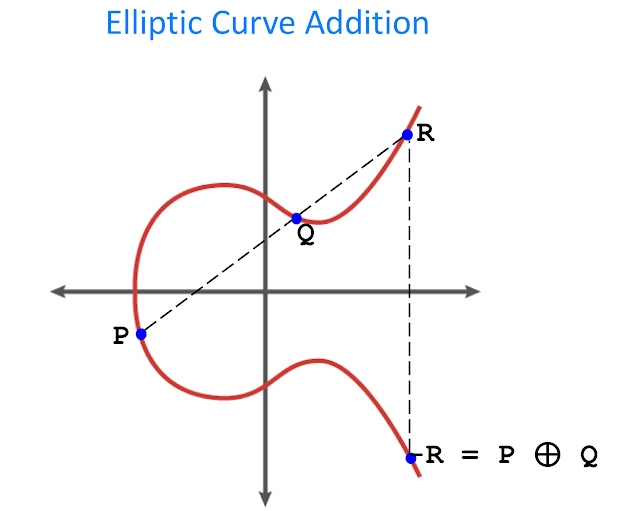Elliptic Curve Points Addition
But wait, what about a scalar point multiplication? Well continuing with the geometric intuition, if P and Q become more and more near (P+Q will be lie 2P) and the line will become a tangent line.Elliptic Curve Point Multiplication
So believe me or not whenever you connect to a website using https (that I hope is the normality in 2019) your browser (and the website server) are actually doing this weird operations describe above just to allow you to have a safe experience (confidentiality of your data, integrity, yadi-yadi-ya). What is important to remember is that both the browser and the server involved in the TLS handshake exchange points in order to agree on a shared secret. But now what? Well here is the bad news called quantum computer. Indeed it turns out that all this beautiful math is easily broken by quantum computer algorithm specifically by Shor's algorithm (in particular this algorithm breaks the Discrete Logarithm Problem on Elliptic Curves aka ECDLP ).  In the course of the last years NIST started a standardisation process with the aim to have a set of algorithms that will be able to resist to the quantum menace. The set of candidates are now reduced to a few instances and the mathematics behind them is the followingMathematics of Post Quantum Cryptography
So as you can see above isogeny-based cryptography is one of the candidates, as you can also read in this excellent blog post from Cloudflare

## Isogeny-based cryptography

So what is an isogeny? I guess it is time to define it. So here we go: an isogeny is a morphism (simply a mapping) described by a rational functions from an elliptic curve E1 to a second elliptic curve E2 that preserves point addition. Now if you remember the previous section about elliptic curve cryptography the gist was that we were exchanging points on a (single) curve. This definition of isogeny hints the fact that for isogeny-based cryptography we might instead "exchanging" curves. Well indeed this is actually the case. But let's slow down and go in order. Actually our isogeny story starts backward in 2006 (I hope you'll understand why later) when Charles, Goren and Lauter defined the CGL hash function. This hash function is a function defined on the graph of supersingular elliptic curves.  A supersingular elliptic curve is a special form of elliptic curve that is a bit "rare" (the "normal" elliptic curve are called ordinary). This supersingular elliptic curves are basically not suitable for standard elliptic curve cryptography (due something called the MOV attack but would take us too afield to describe it). Said that supersingular elliptic curves are the only one that seems to be useful in isogeny based cryptography!! The intuition of CGL was to use isogenies defined on the supersingular domain in order to walk throughout the graph of supersingular elliptic curve: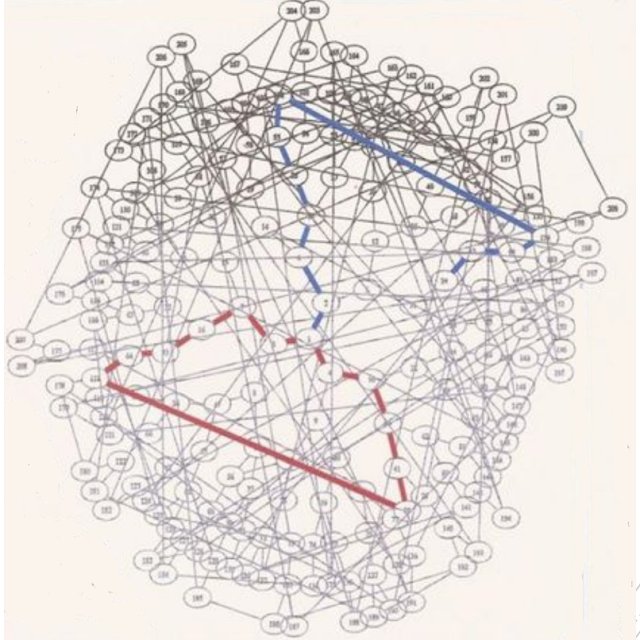CGL hash function
The idea behind CGL is actually pretty neat. Before to describe it we need some notation. Each vertex of the graph above is a different elliptic curve, and each edge is an isogeny between two curves. We also need to mention that in this situation a 2-degree-isogenies (the one used in CGL) starting from a node (elliptic curve) will give you 3 possible routes (isogenies). Apart the starting point we take in consideration only 2 out of this 3 possibilities because we do not want to backtrack (doing this is easy). Last technicality is that  given a supersingular curve defined in the algebraic closure of the finite field GF(p) (with p being prime) hence GF(p²) (remember E is supersingular so the embedding degree is 2), the number of nodes in the above graph will be about p/12 (so in the same magnitude of p). Now suppose we want to hash a binary message M. First define a starting supersingular elliptic curve then for each bit of the message if the bit is equal to 0 we got to one of the 2 not backtracking route and if the bit is equal to 1  we take the other route/isogeny. This way of proceeding has the outcome of having a random walk in this graph (this is a really well known strategy to build hash functions). The already cited paper by Jao and De Feo used the same machinery to build a Diffie Hellman key exchange (SIDH), you can read about it in this excellent blog post. The peculiarity of this kind of construction is that it seems this problem is resistant to attackers that can leverage quantum computer. But what has all this to do with a VDF?

## Verifiable Delay Functions from Supersingular Isogenies and Pairings

We already described VDFs in the previous post where we also mentioned the existence of 3 VDF constructions. If you want to learn about the 2 constructions based on repeated squaring I'd suggest to read this trail of bits blogpost. This post is dedicated to the isogenies VDF. The idea here is to use the same ideas of the CGL hashing function and SIDH to build an efficient VDF. We can try to force the prover to do a huge walk in the graph and then quickly verify that he did not cheat. The walking part is not a big issue (at the end of the day is what both CGL and SIDH do) but what about the verification? This is indeed where the problems start. It is well known that one of the problem that SIDH suffers is the parameter validation during the key exchange as highlighted in this paper. For the record this is why SIDH can be used only with ephemeral keys. So we still need a way to validate. So in order to show you the validation we use in the paper I need to introduce yet another cryptographic tool (the last one I swear) called bilinear pairings. Bilinear pairing is at the basic of yet another branch of cryptography called Pairing-based cryptography. Pairing brought some exiting constructions into the game stuff like Joux's tripartite key exchange or BLS signature. Well there is a nice connections between isogenies and pairing showed here (image taken from Luca De Feo's slides) :Weil pairing and isogenies
Well it seems that this connections might be useful for our VDFs use case. Let's see how:
• Setup: the setup phase of this VDF consists of
1. Choosing a prime number N
2. Selecting a supersingular curve E
3. Performing a random non-backtracking walk of length T having as outcome the isogeny  $$\phi$$ and it's dual $$\hat\phi$$ (every isogeny has a dual isogeny)
4. Choosing a point P and compute $$\phi(P)$$
5. Output  $$\hat\phi,E,E',P,\phi(P)$$
• Eval:
1. Receiving a random point Q
2. Compute $$\hat\phi(Q)$$
• Verify
1. Use the Weil pairing and isogenies formula above to verify that $$\ e_N(P,\hat\phi(Q)) = e_N(\phi(P),Q)$$
It must be noted that the pairing computation in the Verify step is independent from the Eval time T!
This slide taken from Luca De Feo's presenation conveniently summarises everything: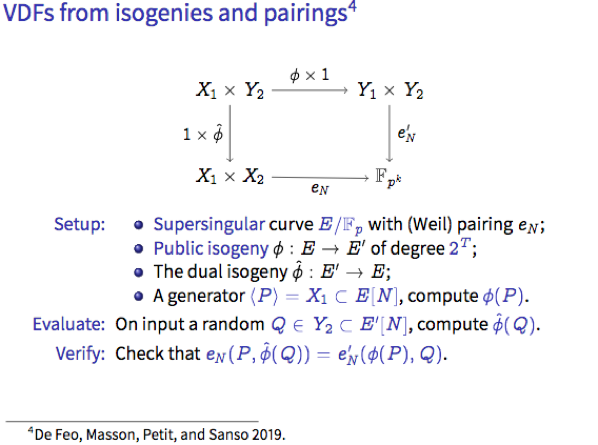Now for the isogenist out there I have to also mention that we defined a VDF also for the supersingular curves defined on GF(p) so without stepping up into the extension field. Now while the VDF's mechanism it might seems similar (and it actually is) the mathematical setting behind the GF(p) case and the GF(p²)  case change drammatically. Indeed while in the GF(p²) case we work on a quaternion algebra, for the GF(p) case we are in the quadratic imaginary field domain. Our VDF construction in the GF(p) case shares similarities with the key exchange protocol CSIDH and with the VDF based on class groups of imaginary quadratic fields by Wesolowski.
Some closing remarks, looking at the VDF comparison table below: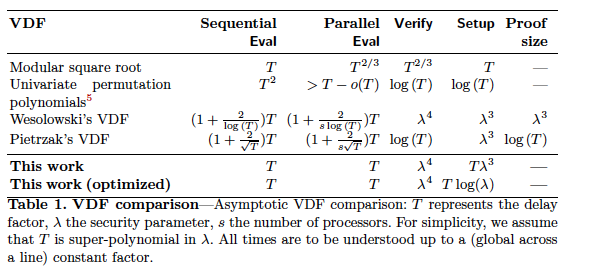VDF comparison
The main advantage of the isogenies VDF is that the the proof is empty, so there is no need to spend extra time to compute it (and store it, did you listen blockchain creators ? you can save space :p ) and as well the Fiat-Shamir transform doesn't need to be part of the game. One other pro about the isogenies VDF is the fact that it is quantum annoying (indeed while this VDF employs isogenies it is not quantum resistant sigh :( due the use of pairings), this means that an adversary armed with a quantum computer needs to break each VDF instance separately. One other thing that the isogenies VDF inherits from BLS signature is the fact that it is also a Verfiable Random Function (VRF) (so not only a VDF but also a VRF). With so many "good features" you would expect there is some little price to pay. This is indeed the case. The main ones are basically two: the setup time is on the same magnitued T as the evaluation (said that this is a one of operation), and as for the other VDFs defined in the RSA groups in order to operate it needs either a trusted setup or a multi-party computation ceremony . Said that at NutMiC 2019 Dan Boneh publicly announced a soon to come paper that might solve the trusted setup issue!

## Conclusions

This is a really high level simplified description of the isogenies VDF. In case you want to look into the details refer to the paper and in case you want to play with it you can find some Sage code in https://github.com/isogenies-vdf/isogenies-vdf-sage (kudos to

## That's all folks! For more Crypto stuff follow me on Twitter.

Antonio Sanso (reporting on joint work with Luca De Feo, Simon Masson, Christophe Petit ).

### OpenSSL Key Recovery Attack on DH small subgroups (CVE-2016-0701)

Usual Mandatory Disclaimer: IANAC (I am not a cryptographer) so I might likely end up writing a bunch of mistakes in this blog post... tl;dr The OpenSSL 1.0.2 releases suffer from a Key Recovery Attack on DH small subgroups . This issue got assigned CVE-2016-0701 with a severity of High and OpenSSL 1.0.2 users should upgrade to 1.0.2f. If an application is using DH configured with parameters based on primes that are not "safe" or not Lim-Lee (as the one in RFC 5114 ) and either Static DH ciphersuites are used or DHE ciphersuites with the default OpenSSL configuration (in particular SSL_OP_SINGLE_DH_USE is not set) then is vulnerable to this attack.  It is believed that many popular applications (e.g. Apache mod_ssl) do set the  SSL_OP_SINGLE_DH_USE option and would therefore not be at risk (for DHE ciphersuites), they still might be for Static DH ciphersuites. Introduction So if you are still here it means you wanna know more. And here is the thing. In my last bl

### Critical vulnerability in JSON Web Encryption (JWE) - RFC 7516

tl;dr if you are using go-jose , node-jose , jose2go , Nimbus JOSE+JWT or jose4j with ECDH-ES please update to the latest version. RFC 7516 aka JSON Web Encryption (JWE) hence many software libraries implementing this specification used to suffer from a classic Invalid Curve Attack . This would allow an attacker to completely recover the secret key of a party using JWE with Key Agreement with Elliptic Curve Diffie-Hellman Ephemeral Static (ECDH-ES) , where the sender could extract receiver’s private key. Premise In this blog post I assume you are already knowledgeable about elliptic curves and their use in cryptography. If not Nick Sullivan 's A (Relatively Easy To Understand) Primer on Elliptic Curve Cryptography or Andrea Corbellini's series Elliptic Curve Cryptography: finite fields and discrete logarithms are great starting points. Then if you further want to climb the elliptic learning curve including the related attacks you might also want to visit https://s

### The Curious Case of WebCrypto Diffie-Hellman on Firefox - Small Subgroups Key Recovery Attack on DH

tl;dr Mozilla Firefox prior to version 72 suffers from Small Subgroups Key Recovery Attack on DH in the WebCrypto 's API. The Firefox's team fixed the issue r emoving completely support for DH over finite fields (that is not in the WebCrypto standard). If you find this interesting read further below. Premise In this blog post I assume you are already knowledgeable about Diffie-Hellman over finite fields and related attacks. If not I recommend to read any cryptography book that covers public key cryptography. Here is a really cool simple explanation by David Wong : I found a cooler way to explain Diffie-Hellman :D pic.twitter.com/DlPvGwZbto — David Wong (@cryptodavidw) January 4, 2020 If you want more details about Small Subgroups Key Recovery Attack on DH I covered some background in one of my previous post ( OpenSSL Key Recovery Attack on DH small subgroups (CVE-2016-0701) ). There is also an academic pape r where we examine the issue with some more rigors.